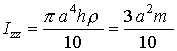EXAMPLE 1: MASS MOMENT OF INERTIA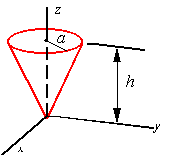Calculate the mass moment of inertia of the cone about the z-axis. Assume the cone is made of a uniform material of density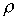(mass per unit volume).

Solution:

The mass moment of inertia about the z-axis is given by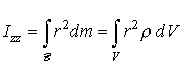The element of volume in a cylindrical coordinate system is given by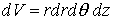#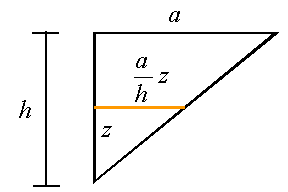The domain of the cone in cylindrical coordinates is defined by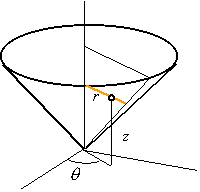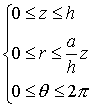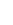Therefore, the mass moment of inertia about the z-axis can be written as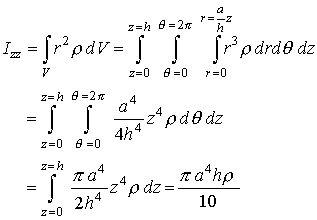For a uniform cone the density can be calculated using the total mass and total volume of the cone so that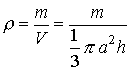Therefore, the moment of inertia in terms of the total mass of the cone can be written as